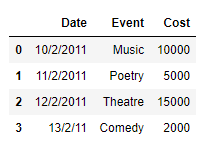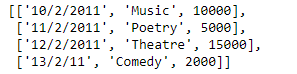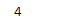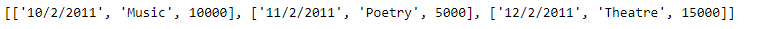# Create a list from rows in Pandas DataFrame | Set 2

In an earlier post, we had discussed some approaches to extract the rows of the dataframe as a Python’s list. In this post, we will see some more methods to achieve that goal.

Solution #1: In order to access the data of each row of the Pandas dataframe, we can use `DataFrame.iloc` attribute and then we can append the data of each row to the end of the list.

 `# importing pandas as pd ` `import` `pandas as pd ` ` `  `# Create the dataframe ` `df ``=` `pd.DataFrame({``'Date'``:[``'10/2/2011'``, ``'11/2/2011'``, ``'12/2/2011'``, ``'13/2/11'``], ` `                    ``'Event'``:[``'Music'``, ``'Poetry'``, ``'Theatre'``, ``'Comedy'``], ` `                    ``'Cost'``:[``10000``, ``5000``, ``15000``, ``2000``]}) ` ` `  `# Print the dataframe ` `print``(df) `

Output :Now we will use the `DataFrame.iloc` attribute to access the values of each row in the dataframe and then we will construct a list out of it.

 `# Create an empty list ` `Row_list ``=``[] ` ` `  `# Iterate over each row ` `for` `i ``in` `range``((df.shape[``0``])): ` ` `  `    ``# Using iloc to access the values of  ` `    ``# the current row denoted by "i" ` `    ``Row_list.append(``list``(df.iloc[i, :])) ` ` `  `# Print the list ` `print``(Row_list) `

Output :As we can see in the output, we have successfully extracted each row of the given dataframe into a list. Just like any other Python’s list we can perform any list operation on the extracted list.

 `# Find the length of the newly  ` `# created list ` `print``(``len``(Row_list)) ` ` `  `# Print the first 3 elements ` `print``(Row_list[:``3``]) `

Output :Solution #2: In order to access the data of each row of the Pandas dataframe we can use `DataFrame.iat` attribute and then we can append the data of each row to the end of the list.

 `# importing pandas as pd ` `import` `pandas as pd ` ` `  `# Create the dataframe ` `df ``=` `pd.DataFrame({``'Date'``:[``'10/2/2011'``, ``'11/2/2011'``, ``'12/2/2011'``, ``'13/2/11'``], ` `                    ``'Event'``:[``'Music'``, ``'Poetry'``, ``'Theatre'``, ``'Comedy'``], ` `                    ``'Cost'``:[``10000``, ``5000``, ``15000``, ``2000``]}) ` ` `  `# Create an empty list ` `Row_list ``=``[] ` ` `  `# Iterate over each row ` `for` `i ``in` `range``((df.shape[``0``])): ` `    ``# Create a list to store the data ` `    ``# of the current row ` `    ``cur_row ``=``[] ` `     `  `    ``# iterate over all the columns ` `    ``for` `j ``in` `range``(df.shape[``1``]): ` `         `  `        ``# append the data of each ` `        ``# column to the list ` `        ``cur_row.append(df.iat[i, j]) ` `         `  `    ``# append the current row to the list ` `    ``Row_list.append(cur_row) ` ` `  `# Print the list ` `print``(Row_list) `

Output :`# Find the length of the newly  ` `# created list ` `print``(``len``(Row_list)) ` ` `  `# Print the first 3 elements ` `print``(Row_list[:``3``]) `

Output :Whether you're preparing for your first job interview or aiming to upskill in this ever-evolving tech landscape, GeeksforGeeks Courses are your key to success. We provide top-quality content at affordable prices, all geared towards accelerating your growth in a time-bound manner. Join the millions we've already empowered, and we're here to do the same for you. Don't miss out - check it out now!

Previous
Next